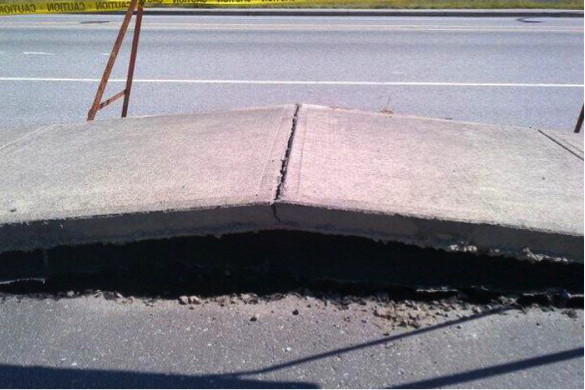## Thermal ExpansionWe didn't do it!

(Source)

When we add heat to objects, the temperature increases. The molecules absorb the heat energy and start not only moving faster, but also farther and farther away from each other. This causes objects to get bigger as their temperature increases. This is why the concrete driveway cracks in the summer (and also why Mama Shmoop won't let us pour boiling water into her fancy glassware).

Solids change in length, in area, and in volume. Makes sense, right? Why would they only expand in one direction?

To calculate the change in length after a given change in temperature, we use the equation LfLi = ΔL = αLiΔT. That's a bit of alphabet soup, so here's the breakdown: Lf is the final length of the object, Li is the initial length of the object, ΔT is the change in temperature, and α is the coefficient of linear expansion of the material.

Every material has a unique coefficient of linear expansion, because different things are made of different molecules and it takes different amounts of heat to excite these different molecules to the same energy level. Increasing a 5 cm long pencil by a millimeter takes a different amount of energy than a 5 cm long rod of Jello. It also takes a different amount of effort to write down your homework answers with them.

How much a material expands also depends on how much the temperature changes. That's why we can pour hot water into mom's fancy glass when the glass is warm, but not when the glass just came out of the freezer. The more the temperature changes, the greater the change in length, and the more likely it is that Mama Shmoop's fine china will become no more.

To calculate the expansion in area or volume of an object we use similar equations to the one for change in length.

AfAi = ΔA = γAiΔT

VfVi = ΔV = βViΔT.

We have γ as the coefficient of area expansion, and β for the coefficient of volume expansion.

One nice thing about these three coefficients is that they aren't completely unrelated to each other for a given substance. Even nicer is that the relationship is easy to remember: the length of an object is one dimensional, its area is two dimensional, and its volume is three dimensional, so we have γ = 2α andβ = 3α.

All right, now it's time to get out of this heat, before we start expanding, too.

### Common mistakes

The three equations for expansion are essentially all the same. If you aren't a walking, talking font of physics knowledge, use the units for area and volume to remember how to convert the coefficients of expansion. Areas have squared units and volumes have cubed units.

To convert the coefficient of linear expansion into the coefficient of area expansion, multiply by 2 (don't square it). Multiply by 3 (no cubing) to get the coefficient of volume expansion.

Okay, you still need to be a spigot of physics knowledge to get this right.